# Water Waves

Glass water tanks are used for examining water waves and its properties. With the help of refraction of light properties of water waves will be explained. You can produce two types of water waves, circular and linear. Picture given below shows how we use light and determine wavelength of the water waves.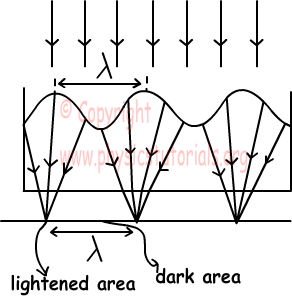Crests of the waves behave like converging lens and we see at the bottom of the tank lightened area. On the contrary, troughs make us see darken area at the bottom of the water tank.

We can create linear waves using a rod, and circular waves using a point source.

Reflection of Linear Water Waves

Linear waves reflect from a linear surface with an angle equal to incident angle. Pictures given below show the incident wave and reflected wave with their angles.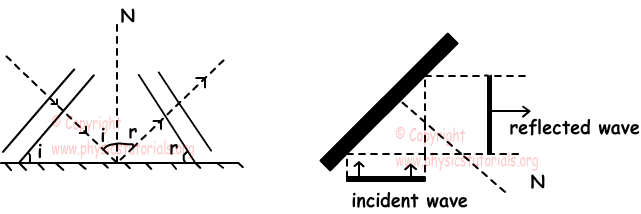Linear waves reflect from a circular surface like in the given picture below. After reflection their shape becomes the reflection surface’s shape.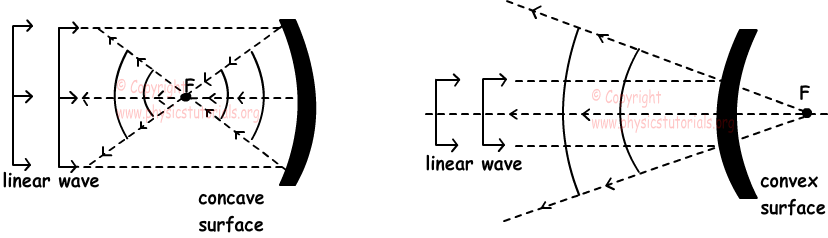In the first picture, waves converge at one point and they turn into circular waves like in the concave mirrors. However, in the second picture, waves reflect from the circular surface as if they are coming from a point behind the surface like in the convex mirrors. Linear waves in the second picture also turn into circular wave.

Reflection of circular wave can be explained like reflection of light from curved mirrors. Pictures given below shows some of the reflection of circular waves.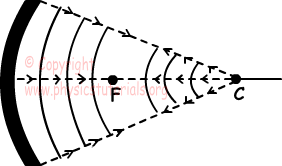Picture given above shows the reflection of circular wave from concave surface. In this picture, waves come from the center of the curvature.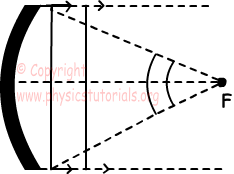Picture given above shows the reflection of circular wave from concave surface. Waves come from the focal point of the surface and reflected circular waves become linear waves.

Waves Exams and Solutions